﻿ Swirling flow through a pipe (1/4 model) - XSim

# Swirling flow through a pipe (1/4 model)

Update: June 1, 2017
OpenFOAM 4.x

## Case directory

\$FOAM_TUTORIALS/incompressible/simpleFoam/pipeCyclic

## Summary

We calculate the case where a swirling flow enters into a cylinder. The fluid flows in from the region "inlet" and out from the region "outlet". The wall of the cylinder is assumed to be a no-slip wall. Only 1/4 of the geometry is modeled, and periodic boundary condition is used on the split planes ("side1" and "side2") to estimate the overall flow.Model geometry (Cross-sectional direction of flow)Model geometry (direction of flow)

In file 0/U, the velocity distribution of the inflow is defined by C++ code using codedFixedValue as follows. That is to say, after calculating the outer product of twice the surface center coordinates and the vector (1, 0, 0), the X component of the calculation result is replaced by 1.0.

```inlet
{
type            codedFixedValue;
name    swirl;

code
#{
const vector axis(1, 0, 0);

vectorField v(2.0*this->patch().Cf() ^ axis);
v.replace(vector::X, 1.0);
operator==(v);
#};
value           \$internalField;
}
```

The calculated velocity distribution is shown below.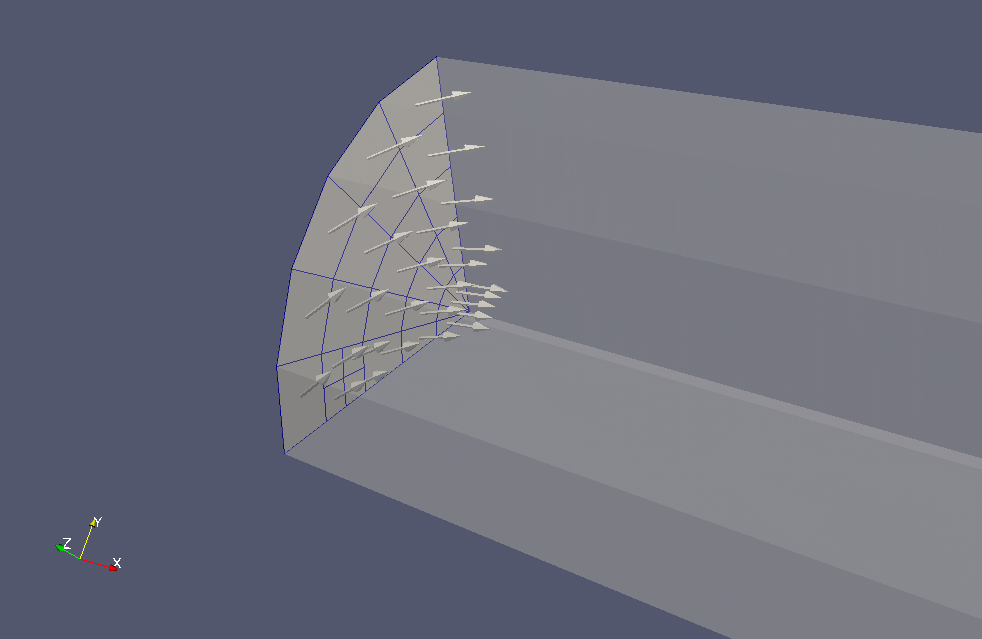Velocity distribution at the inflow region (U)

The meshes are as follows, and the number of mesh is 4100.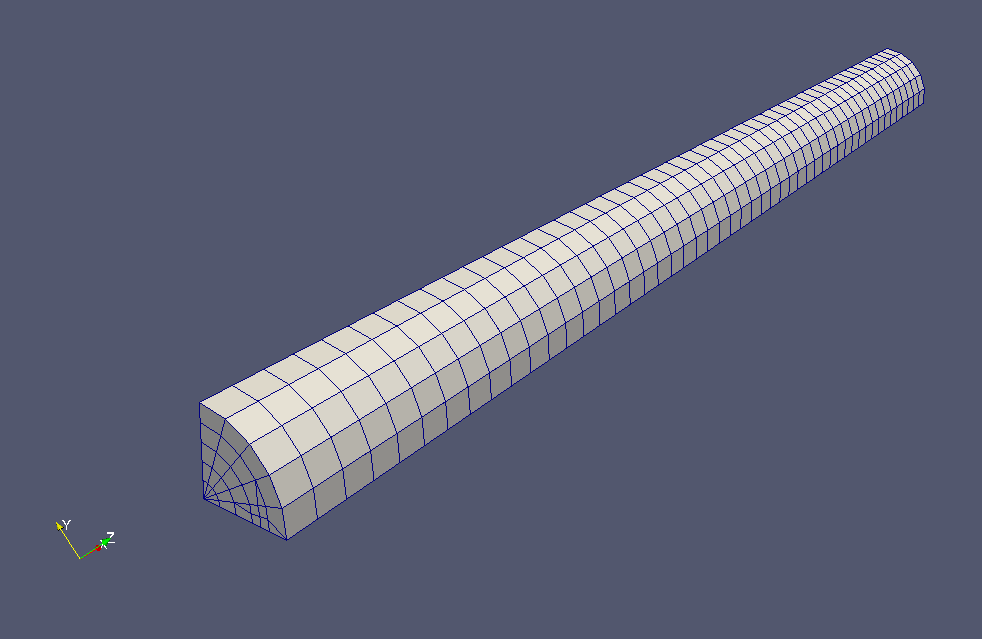Meshes

The calculation result is as follows.Flow velocity in the cross-sectional direction near the center of the pipe (U)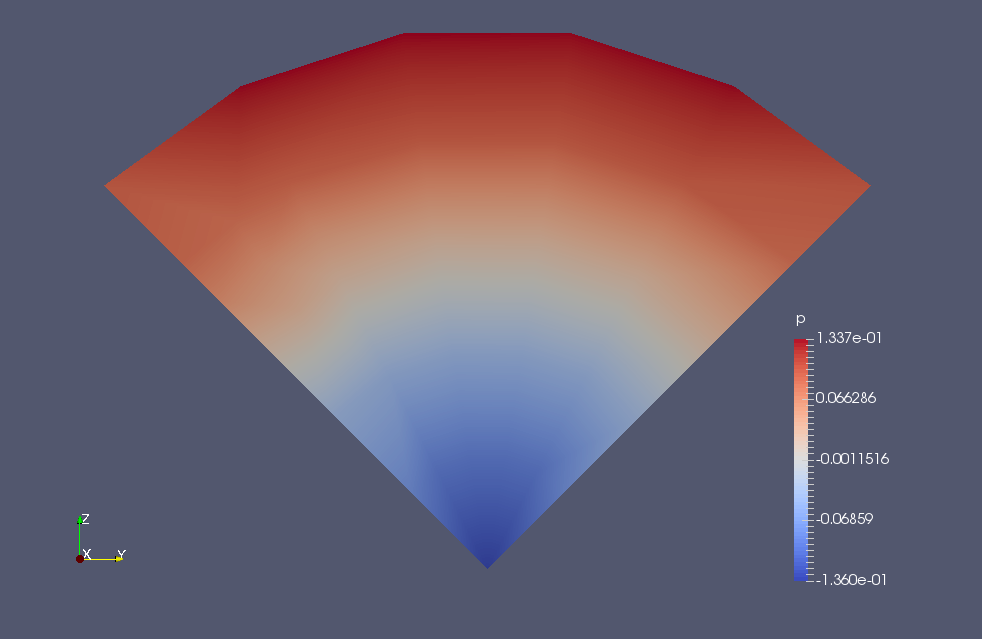Pressure in the cross-sectional direction near the center of the pipe (p)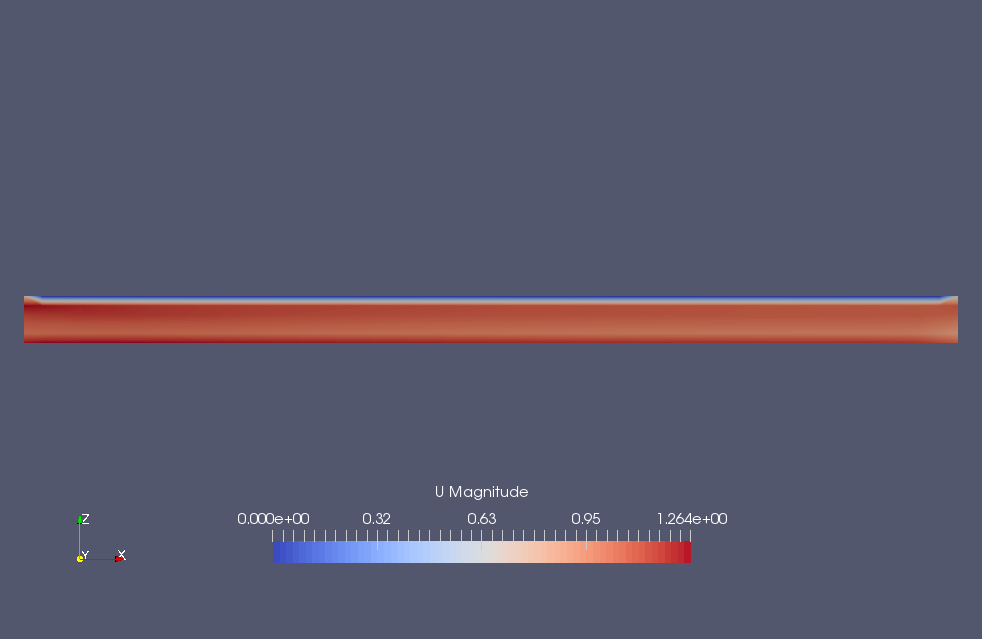Flow velocity in the main flow direction (U)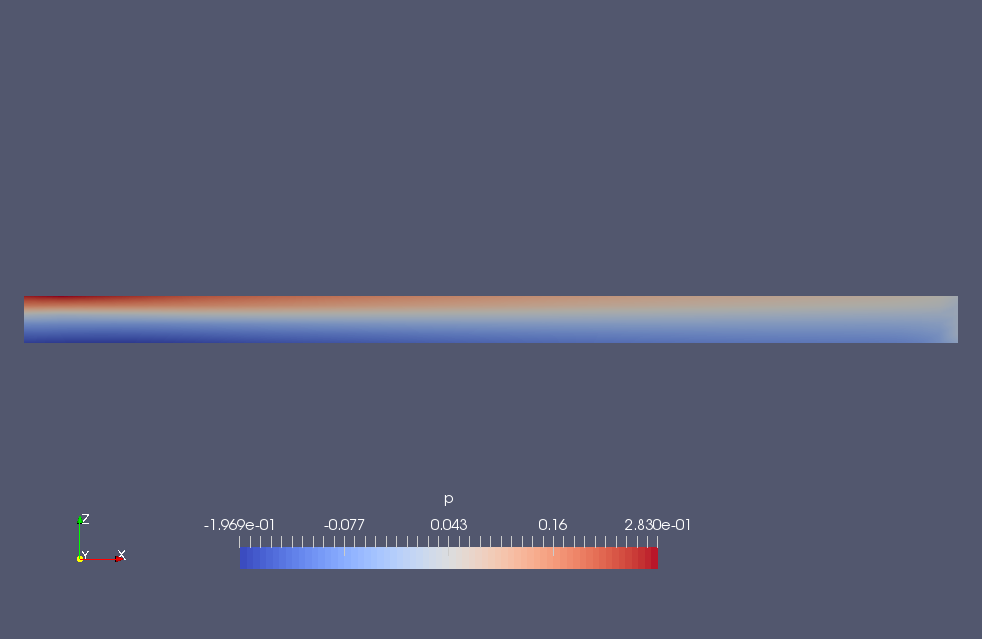Pressure in the main flow direction (p)

## Commands

cp -r \$FOAM_TUTORIALS/incompressible/simpleFoam/pipeCyclic pipeCyclic
cd pipeCyclic

blockMesh
topoSet
refineHexMesh c0 -overwrite
cp -r 0.orig 0

decomposePar -cellDist
mpirun -np 5 simpleFoam -parallel
reconstructPar

paraFoam

In order to check the behavior of the symmetry condition cyclicAMI, we used refineHexMesh to set the number of meshes to be different for the two paired faces.

## Calculation time

• 2.2 seconds *5 parallel, Inter(R) Core(TM) i7-2600 CPU @ 3.40GHz 3.40GHz